# How to Calculate Percentages of Numbers

How to Calculate Percentages of Numbers#### Percentage of a Number Calculator

Enter the percentage and the amount that you wish to calculate the percentage of:

% of

#### How to Calculate a Percentage of any Number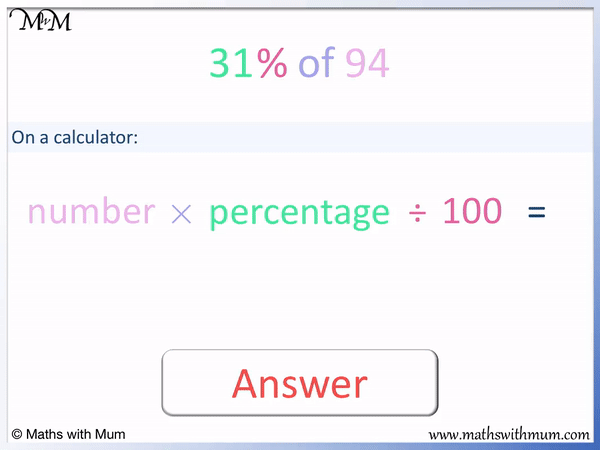• To calculate the percentage of a number, multiply the number by the percentage and then divide by 100.
• The formula for finding a percentage of a number can be written as Number × Percentage ÷ 100 = Answer.
• For example, to find 31% of 94, multiply 94 and 31 and then divide by 100.
• 94 × 31 ÷ 100 = 29.14 and so 31% of 94 = 29.14.
• We divide by 100 because ‘per’ means divide and ‘cent’ means 100. The word ‘of’ means to multiply.
• 31% of 94 means 31 divided by 100 multiplied by 94.
To find a percentage of an amount, multiply the amount by the percentage and divide by 100.

#### Rules to Find Simple Percentages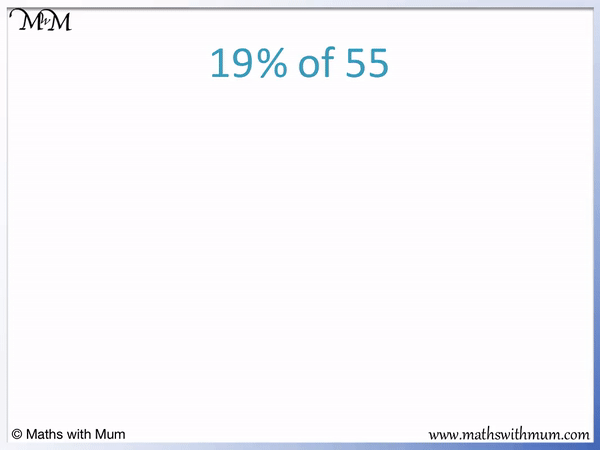• To find a percentage of a number, multiply the number and the percentage and then divide by 100.
• We can use the formula: Number × Percentage ÷ 100 = Answer.
• 55 × 19 ÷ 100 = 10.45.
• 19% of 55 = 10.45.

• We could also work this percentage out by hand.
• 19% is made up of 10% + 9%.
• To find 10%, divide by 10. 55 ÷ 10 = 5.5.
• To find 1% ,divide by 100. 55 ÷ 100 = 0.55.
• To find 9%, multiply 1% by 9. 0.55 × 9 = 4.95.
• To find 19%, add 10% and 9%. 5.5 + 4.95 = 10.45.
•#### Finding Decimal Percentages of Amounts# Calculating Percentages

## How to Calculate Percentages of Numbers

To calculate a percentage of a number, multiply the number by the percentage and then divide by 100. Do not write a percentage sign in your answer. For example, to find 31% of 94, multiply 94 by 31 and then divide by 100. 94 × 31 ÷ 100 = 29.14.The word ‘percent’ means to divide by 100. This is because ‘per’ means divide and ‘cent’ means 100.

In maths, the word ‘of’ means to multiply.

So to find a percentage of a number, we multiply them and also divide by 100.

Here is an example of calculating 19% of 55.To find a percentage of a number, multiply the percentage by the number and then divide by 100.

To find 19% of 55, multiply 19 and 55, then divide by 100.

55 × 19 ÷ 100 = 10.45. Therefore 19% of 55 = 10.45.

This method of finding percentages is best done on a calculator.

## How to Calculate a Percentage with a Calculator

If your calculator has a % key, simply type the percentage followed by the % key and multiply this by the number. If there is no % key on the calculator, multiply the percentage value and the number together but then divide by 100.

Here is an example of finding percentages with a calculator. What is 34.7% of 125?We multiply 125 by 34.7 and then divide by 100.

125 × 34.7 ÷ 100 = 43.375. Therefore 34.7% of 125 = 43.375.

Alternatively, if your calculator has a % button, then we type the following:

34.7% × 125 = 43.375. We do not need to divide by 100 if we have used the % button on the calculator because the % sign does this for us.

The easiest way to find a percentage of a number is to use a calculator. This is because most percentage calculations involve performing a multiplication that is not easy to do without writing down working out.

## Formula to Calculate a Percentage of a Number

The formula to calculate the percentage of a number is Number × Percentage ÷ 100 = Answer. For example, calculating 48% of 64.6, using the formula is 64.6 × 48 ÷ 100 = 31.008.The number is 64.6, the percentage required is 48.

We multiply the two values and then divide by 100.64.6 × 48 ÷ 100 = 31.008 and so 48% of 64.6 is 31.008.

Here is another example of finding a percentage of an amount using the formula.

What is 208% of 700?

Even though the percentage is larger than 100, the rule still applies. The formula can still be used in the same way.The formula to find a percentage of an amount is Number × Percentage ÷ 100 = Answer.

The number is 700 and the percentage is 208.

700 × 208 ÷ 100 = 1456 and so, 208% of 700 = 1456.

The formula of Number × Percentage ÷ 100 = Answer is a good way to find a percentage of an amount when a calculator is available and the numbers involved are larger or contain decimals.

Some percentages are easier to find and can be worked out mentally. These are known as simple percentages.

## Rules to Find Simple Percentages

The following table shows the rules used to find basic percentages of amounts:

Percentage Rule
1% ÷ 100
5% ÷ 20
10% ÷ 10
20% ÷ 5
25% ÷ 4
33.3̄% ÷ 3
50% ÷ 2
75% ÷ 4 then × 3

For example, to find 50% of a number, simply divide it by 2.

50% of 60 means 60 ÷ 2.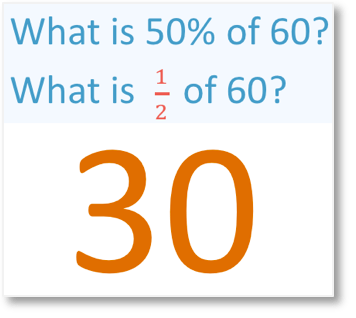60 ÷ 2 = 30 and so, 50% of 60 is 30.

Here is another example of using the rules of finding simple percentages. We are asked to find 25% of 600.To find 25% of a number, divide it by 4.

We can divide by 4 by halving and halving again. Half of 600 is 300 and then half of 300 is 150.

25% of 600 is 150.

The rules to find basic percentages of amounts allow us to find percentages without using a calculator. When introducing percentages, it is worth learning the rules to find simple percentages before trying to find more complicated examples.

Here is a downloadable poster showing some of the ways to find simple percentages:

Here is a list of the rules for finding basic percentages:

• To find 1%, divide by 100.
• To find 5%, divide by 20.
• To find 10%, divide by 10.
• To find 20%, divide by 5.
• To find 25%, divide by 4.
• To find 33.3%, divide by 3.
• To find 50%, divide by 2.
• To find 75%, divide by 4 then multiply by 3.

## Finding Multiples of 10%

To find multiples of ten percent, use the following rules:

Percentage Rule
10% ÷ 10
20% ÷ 10 then × 2
30% ÷ 10 then × 3
40% ÷ 10 then × 4
50% ÷ 10 then × 5
60% ÷ 10 then × 6
70% ÷ 10 then × 7
80% ÷ 10 then × 8
90% ÷ 10 then × 9

We can use these rules to find any multiple of 10 percent.

For example, in this question we are asked, what is 20% of 40?

To find 20%, first find 10% and then double it.

To find 10%, divide by 10.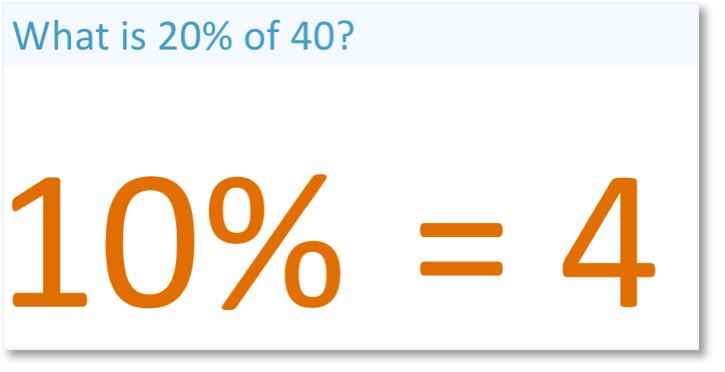10% of 40 is 4.

The next step is to double 10% to find 20%.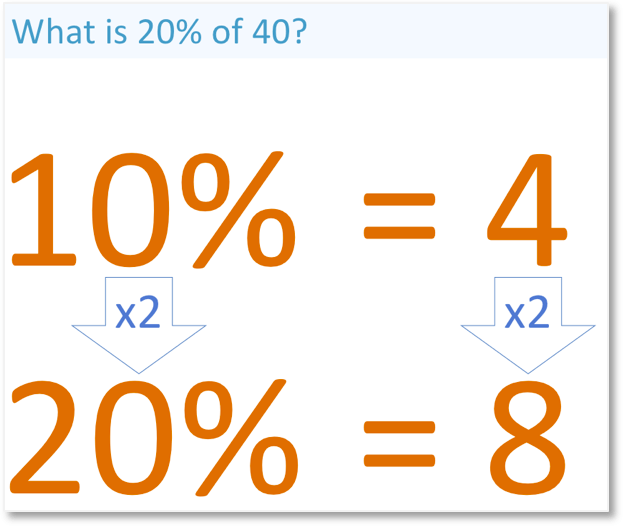20% of 40 is 8.

## How to Calculate a Percentage of a Number without a Calculator

To calculate a percentage of a number without a calculator, follow these steps:

1. Separate the percentage into its tens, ones and decimal place value columns.
2. Divide the number by 10 to find 10% and then multiply this by however many lots of 10% there are.
3. Divide the number by 100 to find 1% and then multiply this by however many lots of 1% there are.
4. Divide the number by 1000 to find 0.1% and then multiply this by however many lots of 0.1% there are.We will start by finding a whole number percentage of an amount. In this example we will find 15% of 40 without a calculator.

The first step is to separate 15% into its tens and ones. 15% = 10% + 5%.To find 10%, divide by 10. 40 ÷ 10 = 4 and so, 10% of 40 is 4.

To find 5%, divide by 20. 40 ÷ 20 = 2. Alternatively, we can find half of 10%. 5% of 40 is 2.

The final step is to add 10% and 5% to make 15%. 4 + 2 = 6, therefore 15% of 40 is 6.

Here is another example of finding any percentage of a number.

What is 35% of 220?The first step is to split 35% into 30% and 5%.

To find 30%, divide by 10 to get 10% and then multiply by 3 to get 30%.

To find 5%, divide by 100 to get 1% and then multiply by 5 to get 5%.

Alternatively, 5% is half of 10%. 10% of 220 is 22 and so, 5% of 220 is 11.The final step is to add the results of 5% and 30% together to find 35%.

30% is 66 and 5% is 11. 66 + 11 = 77 and so, 35% of 220 = 77.Here is an example of finding a percentage of an amount. What is 12% of 200?

The first step is to write 12% as 10% + 2%.

To find 10%, simply divide the number by 10.

To find 2%, find 1% and double it.

To find 1%, divide the number by 100.200 ÷ 10 = 20 and so, 10% of 200 is 20.

200 ÷ 100 = 2 and so 1% of 200 is 2.

Double 1% to find 2%. 2 × 2 = 4 and so 2% of 200 is 4.

The final step is to add 2% and 10% to find 12%.

20 + 4 = 24, therefore 12% of 200 = 24.

In this next example of finding a percentage without a calculator, we have 83% of 1200.

We separate 83% into 80% and 3%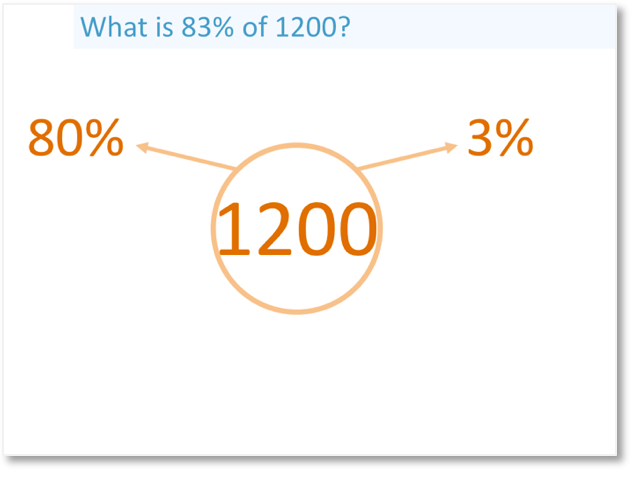To find 80%, find 10% and multiply it by 8.

To find 3%, find 1% and multiply is by 3.1200 ÷ 10 = 120 and so, 10% = 120.

80% = 10% × 8 and so, 80% is 120 × 8. 120 × 8 = 960.

1200 ÷ 100 = 12 and so, 1% = 12.

3% = 1% × 3 and so, 3% is 12 × 3. 12 × 3 = 36.The final step is to add the results together. 80% + 3% = 83%.

80% = 960 and 3% = 36, therefore 960 + 36 = 996.

83% of 1200 = 996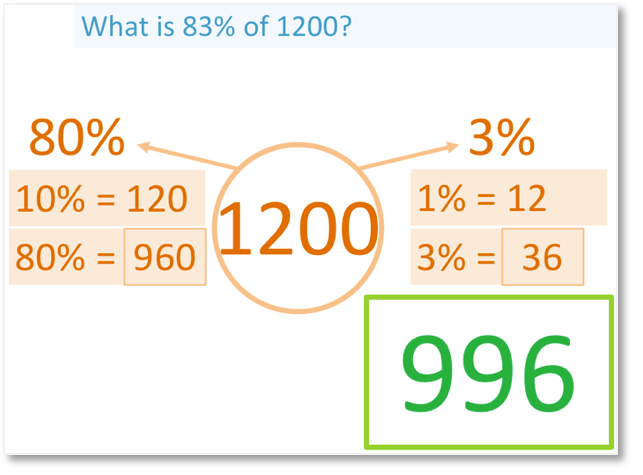When teaching finding percentages of numbers, it is useful to write down the values of all new percentages found as part of the working out. For example, if you have already found 10%, then write this down because we simply need to double it if we need 20% or halve it if we need 5%.

## Examples of Calculating Percentages of Numbers

Here are some further examples of calculating percentages of numbers. In these questions, we will work out the percentages without a calculator.

We will work out how to find the percentage of an amount using examples containing decimal percentages.

What is 23.1% of 2000?

Firstly, separate 23.1% into 20% + 3% + 0.1%.10% is found by dividing the number by 10. 10% = 200.

20% is double 10%. 20% = 400.

1% is found by dividing the number by 100. 1% = 20.

3% is three times 1%. 3% = 60.

0.1% is found by dividing the number by 1000. 0.1% = 2.

We can add up 20% + 3% + 0.1% to find 23.1%.

400 + 60 + 2 = 462 and so 23.1% of 2000 = 462.

In this next question, we will find a decimal percentage of an amount of money.

What is 47.2% of 400?

To find 47.2%, add 40%, 7% and 0.2%.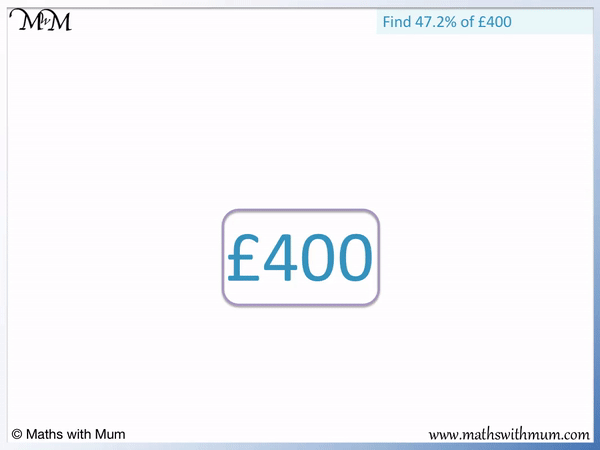To find 10%, divide by 400 by 10. 10% of 400 is 40. To find 40%, multiply 10% by 4. 40% = 160.

To find 1%, divide 400 by 100. 1% of 400 is 4. To find 7%, multiply 1% by 7. 7% = 28.

To find 0.1%, divide 400 by 1000. 0.1% of 400 is 0.4. To find 0.2%, multiply 0.1% by 2. 0.2% = 0.8.

We add 40%, 7% and 0.2% to find 47.2%.

160 + 28 + 0.8 = 188.8. Therefore 47.2% of £400 = £188.80. We write two decimal places with answers involving money.

Here is a question involving finding a percentage of an amount of money in dollars.

What is 84.5% of $1200? We split 84.5% into 80% + 4% + 0.5%.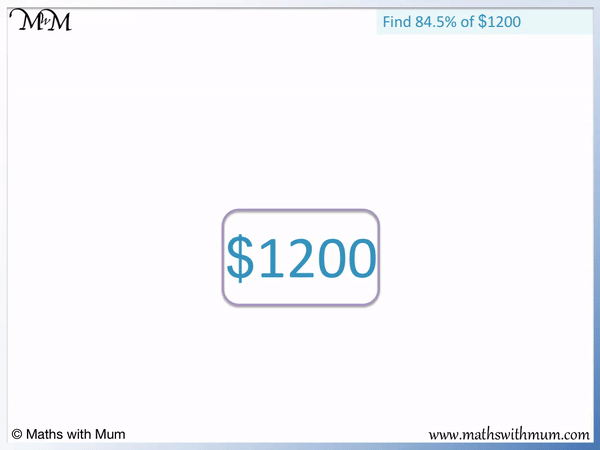To find 10%, divide$1200 by 10.

10% = 120. We multiply this by 8 to get 80%. 80% = 960.

To find 1%, divide $1200 by 100. 1% = 12. We multiply this by 4 to get 4%. 4% = 48. To find 0.1%, we divide$1200 by 1000.

0.1% = 1.2. We multiply this by 5 to get 0.5%. 0.5% = 6. We can also see that 0.5% is half of 1%. 1% = 12 and so, 0.5% is half of 12, which is 6.

Finally, we add 80% + 4% + 0.5% to get 84.5%.

960 + 48 + 6 = 1014. Therefore 84.5% of $1200 =$1014.Now try our lesson on How to Calculate Percentage Increase and Decrease where we learn how to find percentage increase and decrease.error: Content is protected !!